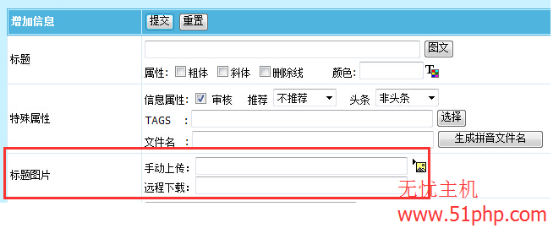1、我们添加字段函数，找到 e/class/userfun.php，打开这个文件，并且将以下代码放入到此文件中：

```function f_picurl(\$mid,\$f,\$isadd,\$isq,\$value,\$cs){
global \$empire,\$dbtbpre,\$public_r;
if(\$value){
return \$value;
}
\$picurl=\$_POST['picurl'];
\$classid=(int)\$_POST['classid'];
if(empty(\$picurl)||!\$classid){
return \$value;
}
\$r=DoTranUrl(\$picurl,\$classid);
if(empty(\$r['tran'])){
return \$value;
}
\$r['filesize']=(int)\$r['filesize'];
\$filepass=(int)\$_POST['filepass'];
return \$r['url'];
}```

2、修改 titlepic 字段，在“后台增加信息处理函数”处填上 f_picurl ，然后修改“输入表单替换html代码”成以下代码：

```手动上传：<input name="titlepic" type="text" id="titlepic" value="<?=\$ecmsfirstpost==1?"":ehtmlspecialchars(stripSlashes(\$r[titlepic]))?>" size="45">
<a onclick="window.open('ecmseditor/FileMain.php?type=1&classid=<?=\$classid?>&infoid=<?=\$id?>&filepass=<?=\$filepass?>&sinfo=1&doing=1&field=titlepic<?=\$ecms_hashur[ehref]?>','','width=700,height=550,scrollbars=yes');" title="选择已上传的图片"><img src="../data/images/changeimg.gif" border="0" align="absbottom"></a>
<br>